### RANDOM Statement

RANDOM random-effects </ options> ;

The RANDOM statement defines the random effects constituting the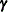vector in the mixed model. It can be used to specify traditional variance component models (as in the VARCOMP procedure) and to specify random coefficients. The random effects can be classification or continuous, and multiple RANDOM statements are possible.

Using notation from the section Mixed Models Theory, the purpose of the RANDOM statement is to define thematrix of the mixed model, the random effects in thevector, and the structure of. Thematrix is constructed exactly as thematrix for the fixed effects, and thematrix is constructed to correspond with the effects constituting. The structure ofis defined by using the TYPE= option.

You can specify INTERCEPT (or INT) as a random effect to indicate the intercept. PROC MIXED does not include the intercept in the RANDOM statement by default as it does in the MODEL statement.

Table 59.15 summarizes the options available in the RANDOM statement. All options are subsequently discussed in alphabetical order.

Table 59.15: Summary of RANDOM Statement Options

Option

Description

Construction of Covariance Structure

Requests that thematrix be read from a SAS data set

Varies covariance parameters by groups

Specifies data set with coefficient matrices for TYPE=LIN

Eliminates columns incorresponding to missing values

Indicates that ratios are specified in the GDATA= data set

Identifies the subjects in the model

Specifies the covariance structure

Statistical Output

ALPHA=Determines the confidence level ()

Requests confidence limits for predictors of random effects

Displays the estimatedmatrix

Displays the Cholesky root (lower) of estimatedmatrix

Displays the inverse Cholesky root (lower) of estimatedmatrix

Displays the correlation matrix corresponding to estimatedmatrix

Displays the inverse of the estimatedmatrix

SOLUTION

Displays solutions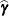of the G-side random effects

Displays blocks of the estimated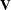matrix

Displays the lower-triangular Cholesky root of blocks of the estimatedmatrix

Displays the inverse Cholesky root of blocks of the estimatedmatrix

Displays the correlation matrix corresponding to blocks of the estimatedmatrix

Displays the inverse of the blocks of the estimatedmatrix

You can specify the following options in the RANDOM statement after a slash (/).

ALPHA=number

requests that a t-type confidence interval be constructed for each of the random-effect estimates with confidence level 1 – number. The value of number must be between 0 and 1; the default is 0.05.

CL

requests that t-type confidence limits be constructed for each of the random-effect estimates. The confidence level is 0.95 by default; this can be changed with the ALPHA= option.

G

requests that the estimatedmatrix be displayed. PROC MIXED displays blanks for values that are 0. If you specify the SUBJECT= option, then the block of thematrix corresponding to the first subject is displayed. For ODS purposes, the name of the table is G.

GC

displays the lower-triangular Cholesky root of the estimatedmatrix according to the rules listed under the G option. The ODS name of the table is CholG.

GCI

displays the inverse Cholesky root of the estimatedmatrix according to the rules listed under the G option. The ODS name of the table is InvCholG.

GCORR

displays the correlation matrix corresponding to the estimatedmatrix according to the rules listed under the G option. The ODS name of the table is GCorr.

GDATA=SAS-data-set

requests that thematrix be read in from a SAS data set. Thismatrix is assumed to be known; therefore, only-side parameters from effects in the REPEATED statement are included in the Newton-Raphson iterations. If no REPEATED statement is specified, then only a residual variance is estimated.

The information in the GDATA= data set can appear in one of two ways. The first is a sparse representation for which you include Row, Col, and Value variables to indicate the row, column, and value of, respectively. All unspecified locations are assumed to be 0. The second representation is for dense matrices. In it you include Row and Col1Coln variables to indicate, respectively, the row and columns of, which is a symmetric matrix of order n. For both representations, you must specify effects in the RANDOM statement that generate amatrix that contains n columns. (See Example 59.4.)

If you have more than one RANDOM statement, only one GDATA= option is required in any one of them, and the data set you specify must contain the entirematrix defined by all of the RANDOM statements.

If the GDATA= data set contains variance ratios instead of the variances themselves, then use the RATIOS option.

Known parameters ofcan also be input by using the PARMS statement with the HOLD= option.

GI

displays the inverse of the estimatedmatrix according to the rules listed under the G option. The ODS name of the table is InvG.

GROUP=effect
GRP=effect

defines an effect specifying heterogeneity in the covariance structure of. All observations having the same level of the group effect have the same covariance parameters. Each new level of the group effect produces a new set of covariance parameters with the same structure as the original group. You should exercise caution in defining the group effect, because strange covariance patterns can result from its misuse. Also, the group effect can greatly increase the number of estimated covariance parameters, which can adversely affect the optimization process.

Continuous variables are permitted as arguments to the GROUP= option. PROC MIXED does not sort by the values of the continuous variable; rather, it considers the data to be from a new subject or group whenever the value of the continuous variable changes from the previous observation. Using a continuous variable decreases execution time for models with a large number of subjects or groups and also prevents the production of a large Class Level Information table.

LDATA=SAS-data-set

reads the coefficient matrices associated with the TYPE=LIN(number) option. The data set must contain the variables Parm, Row, Col1Coln or Parm, Row, Col, Value. The Parm variable denotes which of the number coefficient matrices is currently being constructed, and the Row, Col1Coln, or Row, Col, Value variables specify the matrix values, as they do with the GDATA= option. Unspecified values of these matrices are set equal to 0.

NOFULLZ

eliminates the columns incorresponding to missing levels of random effects involving CLASS variables. By default, these columns are included in.

RATIOS

indicates that ratios with the residual variance are specified in the GDATA= data set instead of the covariance parameters themselves. The default GDATA= data set contains the individual covariance parameters.

SOLUTION
S

requests that the solution for the random-effects parameters be produced. Using notation from the section Mixed Models Theory, these estimates are the empirical best linear unbiased predictors (EBLUPs)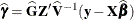. They can be useful for comparing the random effects from different experimental units and can also be treated as residuals in performing diagnostics for your mixed model.

The numbers displayed in the SE Pred column of the Solution for Random Effects table are not the standard errors of thedisplayed in the Estimate column; rather, they are the standard errors of predictions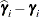, where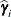is the ith EBLUP and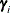is the ith random-effect parameter.

SUBJECT=effect
SUB=effect

identifies the subjects in your mixed model. Complete independence is assumed across subjects; thus, for the RANDOM statement, the SUBJECT= option produces a block-diagonal structure inwith identical blocks. Thematrix is modified to accommodate this block diagonality. In fact, specifying a subject effect is equivalent to nesting all other effects in the RANDOM statement within the subject effect.

Continuous variables are permitted as arguments to the SUBJECT= option. PROC MIXED does not sort by the values of the continuous variable; rather, it considers the data to be from a new subject or group whenever the value of the continuous variable changes from the previous observation. Using a continuous variable decreases execution time for models with a large number of subjects or groups and also prevents the production of a large Class Level Information table.

When you specify the SUBJECT= option and a classification random effect, computations are usually much quicker if the levels of the random effect are duplicated within each level of the SUBJECT= effect.

TYPE=covariance-structure

specifies the covariance structure of. Valid values for covariance-structure and their descriptions are listed in Table 59.17 and Table 59.18. Although a variety of structures are available, most applications call for either TYPE=VC or TYPE=UN. The TYPE=VC (variance components) option is the default structure, and it models a different variance component for each random effect.

The TYPE=UN (unstructured) option is useful for correlated random coefficient models. For example, the following statement specifies a random intercept-slope model that has different variances for the intercept and slope and a covariance between them:

random intercept age / type=un subject=person;


You can also use TYPE=FA0(2) here to request aestimate that is constrained to be nonnegative definite.

If you are constructing your own columns ofwith continuous variables, you can use the TYPE=TOEP(1) structure to group them together to have a common variance component. If you want to have different covariance structures in different parts of, you must use multiple RANDOM statements with different TYPE= options.

V<=value-list>

requests that blocks of the estimatedmatrix be displayed. The first block determined by the SUBJECT= effect is the default displayed block. PROC MIXED displays entries that are 0 as blanks in the table.

You can optionally use the value-list specification, which indicates the subjects for which blocks ofare to be displayed. For example, the following statement displays block matrices for the first, third, and seventh persons:

random int time / type=un subject=person v=1,3,7;


The ODS table name is V.

VC<=value-list>

displays the Cholesky root of the blocks of the estimatedmatrix. The value-list specification is the same as in the V option. The ODS table name is CholV.

VCI<=value-list>

displays the inverse of the Cholesky root of the blocks of the estimatedmatrix. The value-list specification is the same as in the V option. The table name for ODS purposes is InvCholV.

VCORR<=value-list>

displays the correlation matrix corresponding to the blocks of the estimatedmatrix. The value-list specification is the same as in the V option. The table name for ODS purposes is VCorr.

VI<=value-list>

displays the inverse of the blocks of the estimatedmatrix. The value-list specification is the same as in the V option. The ODS table name is InvV.